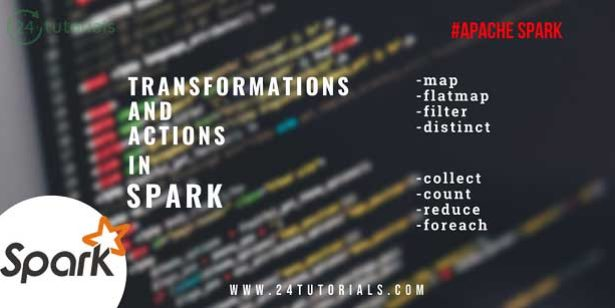Spark

# Transformation and Actions in SparkTransformations and Actions –
Spark defines transformations and actions on RDDs.

Transformations – Return new RDDs as results.
They are lazy, Their result RDD is not immediately computed.
Actions – Compute a result based on an RDD and either returned or saved to an external storage system (e.g., HDFS).
They are eager, their result is immediately computed.
Laziness/eagerness is how we can limit network communication using the programming model.

Example –
Consider the following simple example:
val input: List[String] = List(“hi”,”this”,”is”,”example”)
val words = sc.parallelize(input)
val lengths = words.map(_.length)

Nothing happened on the cluster at this point, execution of map(a transformation) is deferred.
We need to add Action, to kick off the computation and get the results.

val input: List[String] = List(“hi”,”this”,”is”,”example”)
val words = sc.parallelize(input)
val lengths = words.map(_.length)
val totalChars = lengths.reduce(_+_)
println(totalChars)
Result is 15

Some Transformations –

 map map[B](f: A -> B): RDD[B] Apply function to each element in the RDD and return an RDD of the result. flatMap flatMap[B](f: A -> TravarsableOnce[B]): RDD[B] Apply a function to each element in the RDD and return an RDD of the contents of the iterators returned. filter filter(pred: A -> Boolean): RDD[A] Apply predicate function to each element in the RDD and return an RDD of elements that have passed the predicate condition, pred. distinct distinct(): RDD[B] Return RDD with duplicates removed.

Some Actions-

 collect collect(): Array[T] Return all elements from RDD. count count(): Long Return the number of elements in the RDD. take take(num: Int): Array[T] Return the first num elements of the RDD. reduce reduce(op: (A, A) -> A): A Combine the elements in the RDD together using op function and return result. foreach foreach(f: T -> Unit): Unit Apply function to each element in the RDD.